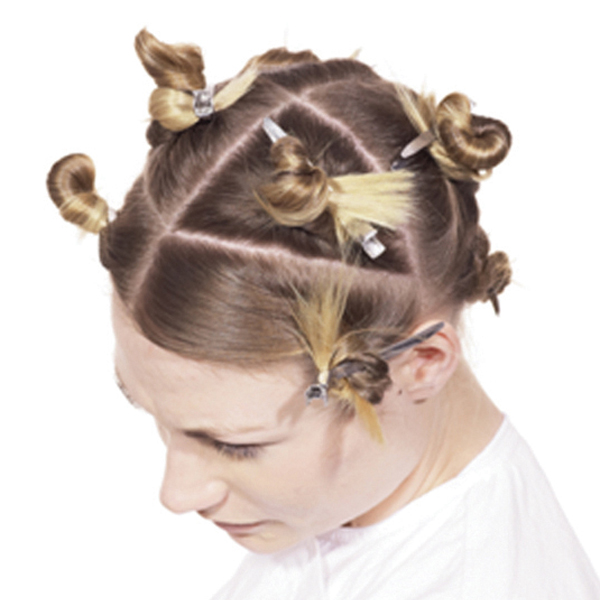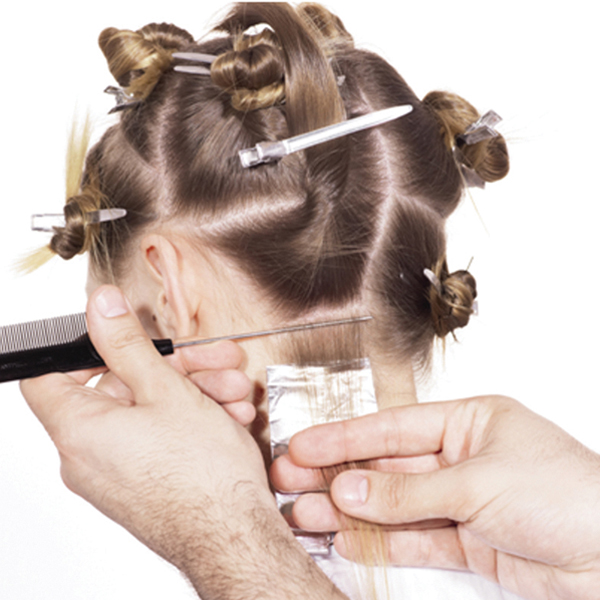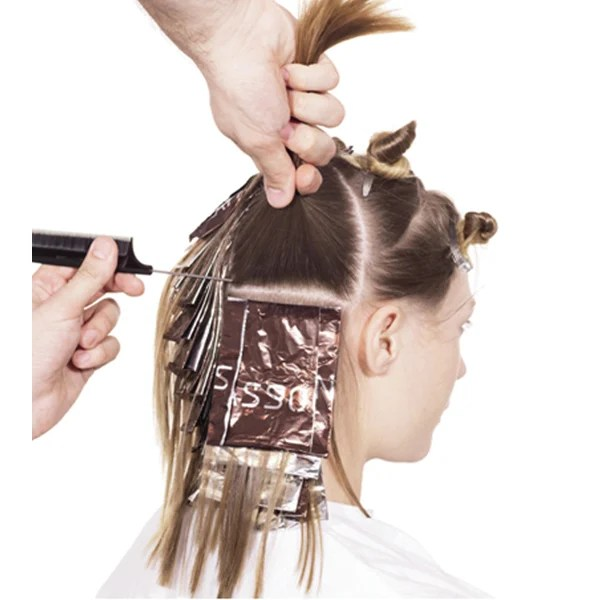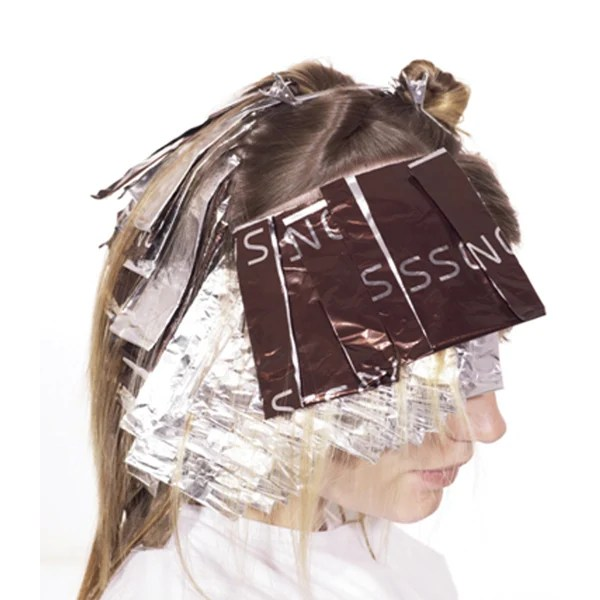Honey Blonde
February 1, 2021

# Honey Blonde

VIEW ALL PHOTOS VIEW SLIDESHOW
GET THE HOW-TO• Formula A (highlights):

Powder lightener + 20-volume developer

• Formula B (highlights):

Pastel blonde or 10N + 40-volume developer

• Formula C (toner):

Equal parts 10N + 9V + 10-volume developer (or comparable demi-permanent formula)# Honey Blonde

For clients who want a natural blonde haircolor, you can never go wrong with a classic honey hue. From sectioning to alternating formula placement, keep scrolling to learn how to achieve this bright blonde shade with subtle hints of dimension!

## COLOR FORMULAS• Formula A (highlights):

Powder lightener + 20-volume developer

• Formula B (highlights):

Pastel blonde or 10N + 40-volume developer

• Formula C (toner):

Equal parts 10N + 9V + 10-volume developer (or comparable demi-permanent formula)

## HOW-TO STEPS•• Formula A (highlights):

Powder lightener + 20-volume developer

• Formula B (highlights):

Pastel blonde or 10N + 40-volume developer

• Formula C (toner):

Equal parts 10N + 9V + 10-volume developer (or comparable demi-permanent formula)

1

Create a large triangular section, placing the widest part of the triangle above the occipital bone and the peak beneath the crown area. Isolate the hairline section, then subdivide the remaining sections—nape, occipital to crown, crown to front hairline and hairline—creating smaller triangular and rectangular sections.

•• Formula A (highlights):

Powder lightener + 20-volume developer

• Formula B (highlights):

Pastel blonde or 10N + 40-volume developer

• Formula C (toner):

Equal parts 10N + 9V + 10-volume developer (or comparable demi-permanent formula)

2

Starting in the nape section, take a horizontal weave and apply Formula A. Fold the foil gently in two without any tension to avoid pulling away from the root. Then, take a slice above that first section and apply Formula B. Continue alternating between the two formulas as you work your way up the head.

•• Formula A (highlights):

Powder lightener + 20-volume developer

• Formula B (highlights):

Pastel blonde or 10N + 40-volume developer

• Formula C (toner):

Equal parts 10N + 9V + 10-volume developer (or comparable demi-permanent formula)

3

As the section becomes two foils wide, be sure to place the same formula in the adjacent foil. Once you reach the top of the head, the foil will take on a more triangular shape, allowing it to stay closer to the root area. For a softer result at the crown, the last two foils are weaved, not sliced.

•• Formula A (highlights):

Powder lightener + 20-volume developer

• Formula B (highlights):

Pastel blonde or 10N + 40-volume developer

• Formula C (toner):

Equal parts 10N + 9V + 10-volume developer (or comparable demi-permanent formula)

4

Repeat the same steps on the opposite side of the head, making sure to always apply the same formulas adjacent to each other.

•• Formula A (highlights):

Powder lightener + 20-volume developer

• Formula B (highlights):

Pastel blonde or 10N + 40-volume developer

• Formula C (toner):

Equal parts 10N + 9V + 10-volume developer (or comparable demi-permanent formula)

5

In the front hairline section, switch to a back-to-back slicing technique. To create a more solid effect through the front, do not leave any hair out between the foils. Also, avoid placing color on the roots.

•• Formula A (highlights):

Powder lightener + 20-volume developer

• Formula B (highlights):

Pastel blonde or 10N + 40-volume developer

• Formula C (toner):

Equal parts 10N + 9V + 10-volume developer (or comparable demi-permanent formula)

6

In the section behind the back-to-back panel, resume the regular slicing technique. Once again, hair is left out between the foils.

•• Formula A (highlights):

Powder lightener + 20-volume developer

• Formula B (highlights):

Pastel blonde or 10N + 40-volume developer

• Formula C (toner):

Equal parts 10N + 9V + 10-volume developer (or comparable demi-permanent formula)

7

Return to the back-to-back panel. Pulling the foil away, apply Formula A to the root and process to the desired lift before rinsing.

•• Formula A (highlights):

Powder lightener + 20-volume developer

• Formula B (highlights):

Pastel blonde or 10N + 40-volume developer

• Formula C (toner):

Equal parts 10N + 9V + 10-volume developer (or comparable demi-permanent formula)

8

Re-section the hair, isolating the back-to-back hairline section. Then, subdivide the section at the center and apply Formula C to tone, extending the application down through the sides.

•• Formula A (highlights):

Powder lightener + 20-volume developer

• Formula B (highlights):

Pastel blonde or 10N + 40-volume developer

• Formula C (toner):

Equal parts 10N + 9V + 10-volume developer (or comparable demi-permanent formula)

9

Isolate these sections in foil and process according to manufacturer’s instructions.

•• Formula A (highlights):

Powder lightener + 20-volume developer

• Formula B (highlights):

Pastel blonde or 10N + 40-volume developer

• Formula C (toner):

Equal parts 10N + 9V + 10-volume developer (or comparable demi-permanent formula)

10

Finished look